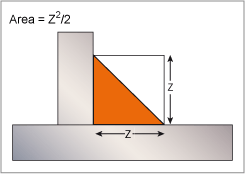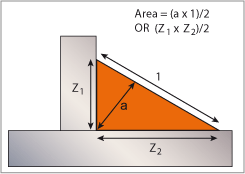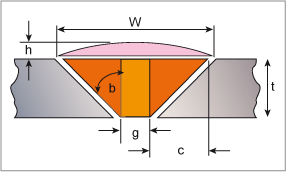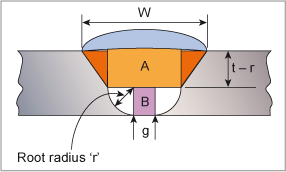Subscribe to our newsletter to receive the latest news and events from TWI:

# Calculating weld volume and weight

Calculating the volume of a weld is one of the first steps to be taken when estimating the cost of making a weld.

With this information, and knowing the deposition rate of the process, it is possible to determine the arc time (the length of time that an arc is burning and depositing weld metal) and the amount of welding consumables required to fill the joint. Both of these are required in order to calculate the cost of making the weld. Costing will be dealt with in future Job Knowledge articles.

##Determining the volume of a weld requires some knowledge of basic geometrical calculations to determine the area of the weld and multiply this figure by its length. The first step then is to calculate the cross sectional area of the joint.

With a fillet weld or a 45° single bevel joint this is relatively simple but the calculations become lengthier as the weld preparation becomes more complex. Fig.1 illustrates how simple this calculation is for an equal leg length fillet weld; the area of such a weld is half the square of the leg length, Z. When using this formula do not forget that welders seldom deposit precisely the size of weld called up on the drawing or in the welding procedure and that there may be some excess weld metal on the face of the weld.Fig.1. Area of an equal leg length fillet weld

An asymmetrical fillet weld is a little more difficult; the area of a triangle is given by the base Z2 times the height Z1 divided by 2 so when a fillet weld is deposited with unequal leg lengths the area can be calculated from multiplying the throat, a, by the length of the face I and divided by 2 as illustrated in Fig.2.Fig.2. Area of an unequal leg length fillet

Turning now to butt welds, the calculations become a little more complex.

There are three factors that determine the volume of the weld in a single V butt weld. These are the angle of the bevel, b, the excess weld metal and the root gap, g, as illustrated in Fig 3. To calculate the area of this weld we need to be able to add together the areas of the four components illustrated in Fig.3.Fig.3. The four areas of a single-V butt weld

The dimension 'c' is given by (tan b x t); the area of a single red triangle is therefore t(tan b x t)/2. The total area of the two red regions added together can be calculated using the formula 2t(tan b x t)/2 or t(tan b x t).

The width of the weld cap, w, is given by W = 2(tan b x t) + g.

The area of the excess weld metal is approximated by the formula (W x h)/2.

The area provided by the root gap by g x t.

The bevel angles, b, most often used are 10° = (tan 0.176), 15° = (tan 0.268), 22.5° = (tan 0.414) 32.5° = (tan 0.637) and 45° = (tan 1.00). As will become obvious when the weight is calculated, it is easier to ensure that the decimal point is in the right place if centimetres are used in the calculations rather than millimetres.

As a worked example, if the weld is in a plate 2.5cm thickness, 0.3cm root gap, 65° included angle (b = 32.50°; tan 32.5° = 0.637) and with a cap height of 0.2cm we have:-

1. c = tan32.5 x 2.5 = 0.637 x 2.5 = 1.59cm
2. w = 2(0.637x2.5) + 0.3 = 3.485cm so the area of the cap = (3.485x0.2)/2 = 0.348 sq. cm.
3. area of the orange area = 0.3 x 2.5 = 0.75 sq.cm.
4. area of the two red areas = 2 x (1.59 x 2.5)/2 = 3.97sq.cm.

This gives a total area of 5.07sq cm. The volume can then be calculated by multiplying the length of the weld by the area - ensuring that this length is also given in centimetres!

Conventionally, the volume is often expressed in cubic centimetres (cu.cm). per metre so in this example the volume is 507 cu. cm/metre.

To obtain the weight of weld metal this figure is then multiplied by the density of the alloy. Table 1 gives the density of some of the more common alloys in gm/cu.cm. Note that with some alloys the alloying elements can change the density quite significantly.

Table 1. Densities of some of the more common alloys.

AlloyDensity (gm/cm3)
iron 7.87
0.25% carbon steel 7.86
12%Cr steel 7.70
304 stainless steel 7.92
nickel 8.90
80/20 Ni.Cr 8.40
625 type alloy 8.44
copper 8.94
70/30 brass 8.53
7% Al bronze 7.89
aluminium 2.70
Al 5052 2.65
Al 7075 2.8

The weight of weld metal to fill one metre length of the joint described above would therefore be; in carbon steel (507 x 7.86) = 3985gms or 3.98kgs/metre; in a 5XXX series aluminium alloy (507 x 2.65) = 1343gms, 1.34kgs/metre.

Calculating the weight of weld metal in double sided V-joints uses the same approach by dividing the weld into its individual 'V's and adding the products.

A J-preparation, however, adds another area into the equation; that of the half circle at the root of the weld, see Fig.4. The formulae given above to calculate 'c', the area of the two red components and the excess weld metal remain unchanged but the width of the cap must be increased by 2r. There are also the two areas, 'A' and 'B', to calculate and the two white root radius areas to be added to the total.Fig.4. Single 'U' preparation (other notation as in Fig.3)

The relevant formulae are thus:

1. the dimension 'c' is given by (tan b x (t-r)); the total area of the two red regions is therefore given by the formula 2((t-r)(tan b x (t-r))/2 or ((t.-r)(tan b x (t-r)).
2. the width of the weld cap, w, is given by w = 2(tan b x (t-r)) + g +2r.
3. the area of the excess weld metal is given by the formula (w x h)/2.
4. the area 'A' is (t-r) x (2r +g).
5. the area 'B' is g x r.
6. the root radius area is (πr2)/4

For a double-U preparation it is necessary to calculate the areas of both sides and add these together.

Having calculated the weight of weld metal required to fill a weld preparation it is then possible to calculate the weight of filler metal required (these two figures are not necessarily the same) and to estimate the time required to deposit this weld metal; both essential in order to arrive at a cost of fabricating the weld. This will be covered in future Job Knowledge articles.